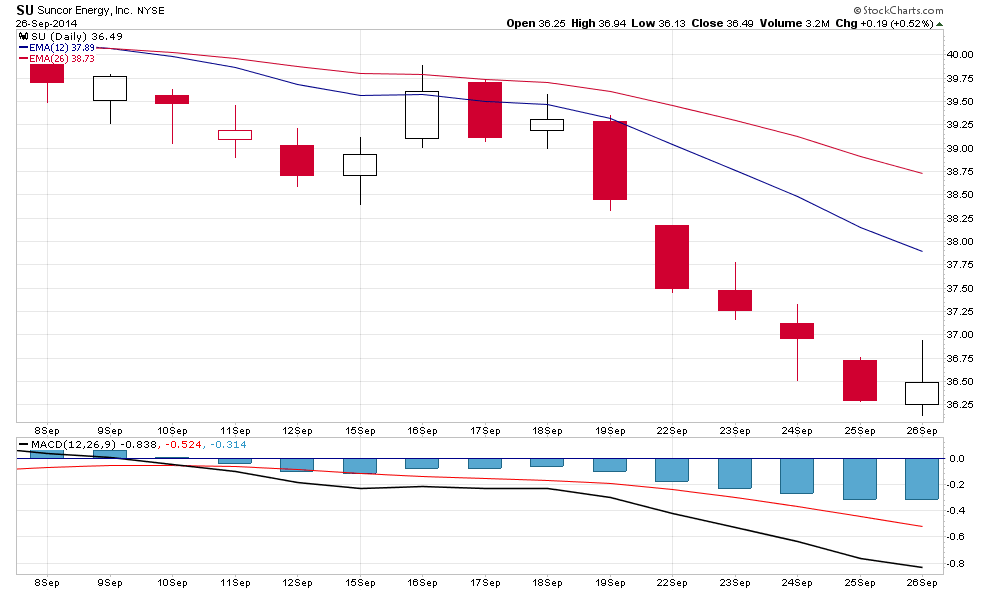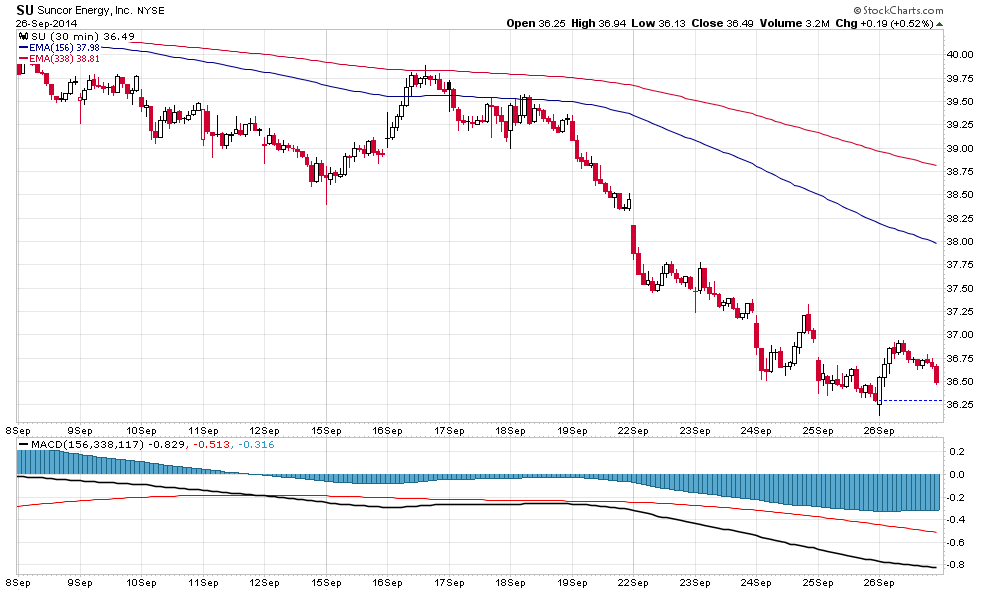#### Howdy, Stranger!

It looks like you're new here. Sign in or register to get started.

# What intraday period does SCC use to calculate indicator values on the 60 minute intraday chart?

Question: What intraday period does SCC use to calculate indicator values on the 60 minute intraday chart?

Hi all,

I am curious to know what intraday period SCC uses to calculate indicators on 60 minute charts? I figure that on Daily charts, daily periods are used to calculate indicators. Daily calculations would not need the intraday periods. Correct? Weekly calculations use the daily periods and would not need intraday periods. Correct? So, to build the 60 minute chart, what intraday period is used?

Thanks for help on this. Getting deep on the questions.• edited September 2014 Answer ✓
Kevo, I suggest you read some of the ChartSchool training documents, they explain all the indicators along with applications and how they are calculated, with Excel spreadsheet examples.

With respect to your comments, you are partially right and partially wrong.

Every indicator is calculated using the bar period of the chart. For example MACD on a daily chart uses the closing daily values of each daily bar to calculate the EMA's used in the MACD. As these are EMA's rather than SMA's, the more recent values are more heavily weighted in the average than are the earlier ones. If we were looking at say Bollinger Bands which use SMA's then all values are equally weighted in the calculation.

Now would using 30 minute bars, (intraday values) give you a more accurate value of the daily MACD. Well not really as you are now not calculating based on the closing values, but using intraday values.

If you plot MACD (12,26,9) on the daily, you are looking at the last 12 and 26 day EMA's. If you use the same MACD (12,26,9) on a 30 min chart, then you are only looking at the last 12 and 26 bars, which is basically the last 1 & 2 days. IE one day has 13, 30 minute bars. So to get a 12 day range you would have to use 12 X 13 = 156 bars at 30 min ea. similar for the 26 and 9 averages.

So you are really looking at two different things, although in some cases they will yield similar values, in other cases they can be quite different. See the following charts.• The best way is not to think about specific time periods. IE the SMA-20 of the close is the 20 PERIOD or 20 BAR average. So on a daily chart it's 20 daily bars, on a weekly chart its 20 Weekly bars, on a 60 min chart its 20, 60 min bars.
• Thanks gord. I think I miss-stated my question, and I did not ask my question properly.

I mean indicator values like Stochastics, MACD, CCI, and TRIX. To get more accurate values for these, would a smaller period like 5 min or 1 min be used to calculate this, or can accurate values be produced by using 60 min bars on a hourly chart? Would 30 minute bars make a difference?

Did I just answer my own question? If daily bars are used to calculate MACD and CCI on daily charts, then 60 minute bars are used to calculate MACD and CCI on hourly charts?

So does this mean that on Weekly charts, Weekly bars are used to calculate MACD and CCI - not Daily bars?

I guess what I am trying to ask is: is there no difference between using 1, 5, 30, or 60 minute bars to build the indicators like MACD and CCI that show up on an hourly chart?

Thanks.
• Very nicely said gord. Thank you. I have made note of your information.I think I need to come clean on why I started this post.I recently started using a test system where I can load 1, 5, 15, or 30 minute bars and then build 60 minute charts. I noticed some discrepancies after comparing with SCC. I am only experimenting and learning with this system. The mistake I made is that any questions or related questions about this system, I need to refer to that provider's support. I started this post based on questions I had about that system. That was the mistake I made. I will be cognizant of situations like this going forward.

This turned out to be a good learning experience because of the mistake and because I see that since 1 minute bars is the smallest period, all indicators on the 1 minute bar chart use the closing price on the 1 minute bars for its calculation as you explain above. The closing price on the 1 minute bar is all that is needed since we are only looking at that smaller scale. For larger scales (or periods), I figure that the 1 minute bars are converted to 5 minute, 30 minute, hourly, daily, weekly bars, etc. I presume that SCC would only need to obtain 1 minute bars from the data provider because SCC can then convert the 1 minute period to larger periods to then be used in the respective indicator calculations for larger periods.